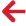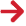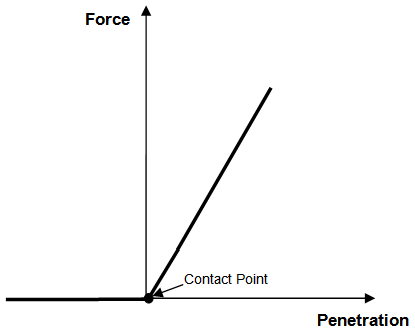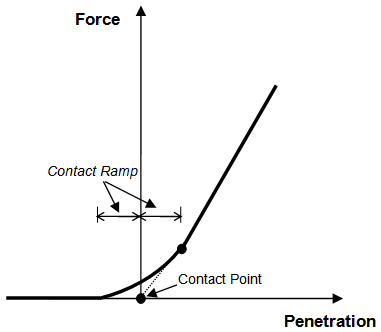﻿ PipeLay > Technical Notes > Technical Note 5 - Contact Modelling Options > Contact Modelling Strategy

# Contact Modelling StrategyFor each of the contact modelling options, the most important aspect of the contact algorithm is how PipeLay handles the interaction between the pipeline and the rollerbox surfaces in the direction normal to each surface. The approach used is basically similar for each of the modelling strategies, and differs fundamentally from that used in earlier versions of PipeLay. This section describes how the contact algorithm now functions.

Each node on the pipeline that is potentially in contact with a rollerbox may be considered to be connected to each surface of the rollerbox by means of a non-linear spring that has an orientation normal to the surface. Theoretically, each of these springs should have the force-deflection (or penetration) characteristics shown in Figure TN.5.1, whereby the force in the spring is zero until the node makes contact with the surface, at which point the linear spring stiffness is activated. While this formulation is theoretically correct, it is not especially robust, so PipeLay uses the spring force-deflection curve shown in Figure TN.5.2 instead.Figure TN.5.1: Therotical Force-Deflection Characteristic for Contact SpringFigure TN.5.2: PipeLay Force-Deflection Characteristic for Contact Spring

In Figure TN.5.2, rather than the contact spring stiffness being activated suddenly when contact occurs, the force in the spring is gradually ‘ramped up’. This means that there is some force in the spring before a node penetrates the rollerbox surface, but the magnitude is relatively small. Overall, the contact algorithm provides a robust and accurate engineering approximation. The distance over which the force in the spring is ramped up is symmetric about the Force axis. In other words, the distance from the start of the ramp to the Force axis is the same as the distance from the Force axis to where the ramp meets the linear surface stiffness curve. In PipeLay, these equal distances define an input called the Contact Ramp in the Project component. The Contact Ramp defaults to 0.1m or 0.328ft. This means that the overall ramp length is 0.2m or 0.656ft by default. To change the contact ramp, you can use the Contact Ramp input on the Project component. If you set the Contact Ramp to zero, then the spring force-deflection characteristics are as shown in Figure TN.5.1.

It is also worth noting that the curve used to gradually ramp up the force in the contact spring is governed by a power law equation and the associated ‘power’ value can be specified through the Contact Power input on the Project component. The default value for this input is 1.5, which represents an optimum power for low to medium stiffness supports. However for high stiffness supports (> 50000 kN/m or 3400 kips/ft) you may wish to reduce the Contact Power to a smaller value, say 1, so as to achieve optimum run times. By reducing the power the curvature of the ramp curve decreases whereas the opposite occurs when you increase the power.